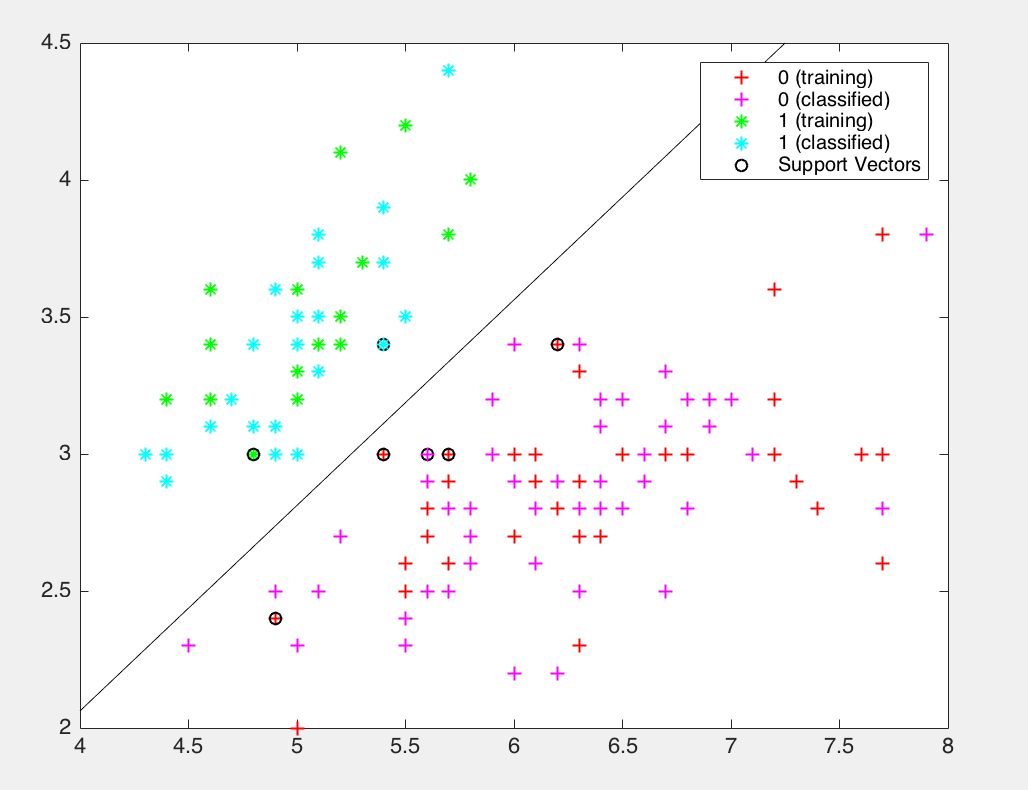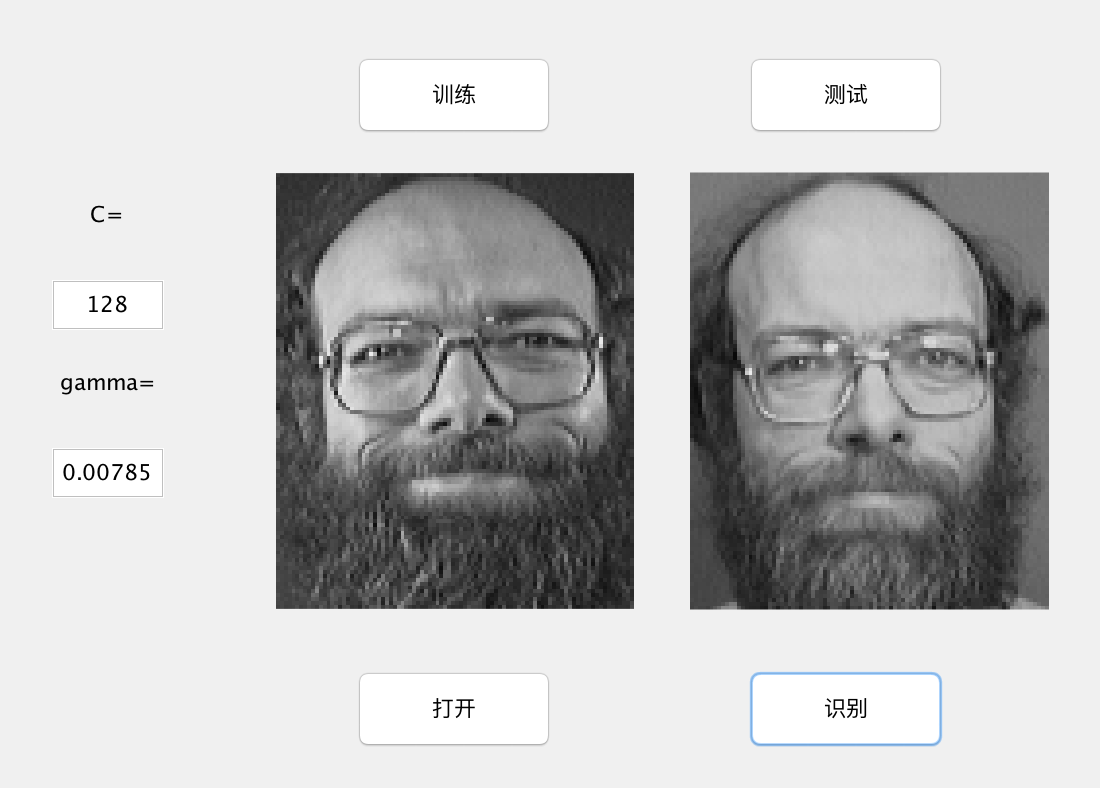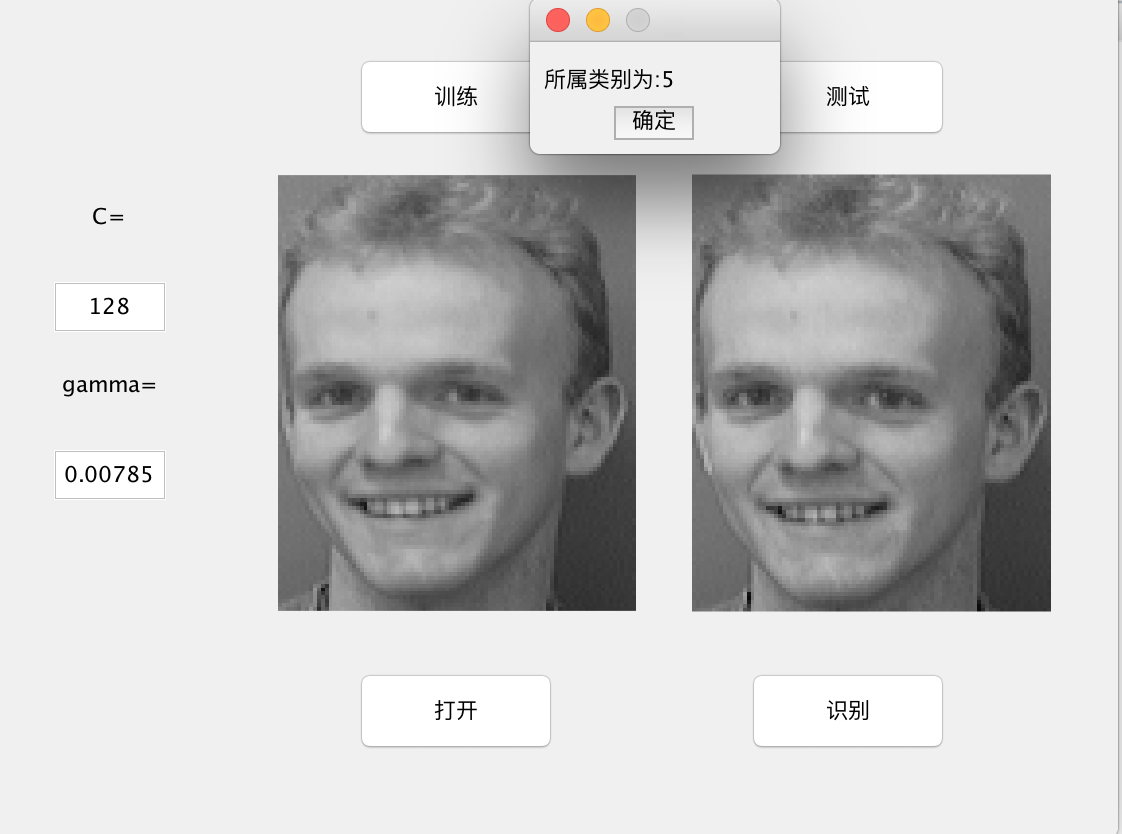# 基于PCA和SVM的人脸识别

Posted by jjx on January 7, 2017

#### svm推广到多类情况

• A. 所对应的样本特征向量作为正集（类标签为+1), B、C、D所对应的样本特征向量作为负集（类标签为-1).
• B所对应的样本特征向量作为正集，A. C. D所对应的样本特征向量作为负集
• C所对应的样本特征向量作为正集， A. B, D所对应的样本特征向量作为负集．
• D所对应的样本特征向量作为正集， A. B、 C所对应的样本特征向量作为负集．

3. 一对一的淘汰策略(one against one with. eUmlnating)

(1) 设被识别对象为X, 首先由1#判别函数进行识别。若判别函数h(x) = +1, 则结果为类型A, 所有关于类型C的判别函数均被淘汰；若判别函数h(x) =-1, 则结果为类型C,所有关于类型A的判别函数均被淘汰；若判别函数h(x) = 0, 为“ 拒绝决策” 的情形， 则直接选用2#判别函数进行识别。
(2)被识别对象x再由4#判别函数进行识别。若结果为类型+1, 淘汰所有关于D类的判别函数， 则所剩判别函数为6# (B,C)。
(3) 被识别对象x再由6#判别函数进行识别。若得到结果为类型+1 则可判定最终的分类结果为B。

#### SVM的Matlab实现

SVMStruct = svmtrain(Tranining,Group);
• Training是一个包含训练数据的m行n列的2维矩阵。每行表示1个训练样本（特征 向量），m表示训练样本数目； n表示样本的维数．
• Group是一个代表训练样本类标签的1维向量．其元素值只能为0或1.通常1表示正例，0表示反例.Group的维数必须和Traningg的行数相等，以保证训练样本同其 类别标号的一一对应．
SVMStruct是训练所得的代表SVM分类器的结构体，包含有关最佳分割超平面的种种信息，也可以计算出分类间隔值。

svmtrain(…,”ShowPlot”,true);

svmStruct = svmtarin(…,”boxconstraint”,C);

Group = svmclassify(SVMStruct,sample);
SVMStruct是训练得到的代表SVM分类器的结构体， 由函数svmtrain返回．
Sample是要进行分类的样本矩阵， 每行为1个样本特征向量，总行数等于样本数目，总列数是样本特征的维数，它必须和训练该SVM时使用的样本特征维数相同．Group是一个包含Sample中所有样本分类结果的列向量， 其维数与Sample矩阵的行数相同．

svm训练分类鸢尾植物

load fisheriris;
data = [meas(:,1),meas(:,2)];
groups = ismember(species,'setosa');

[train,test] = crossvalind('holdOut',groups);

svmStruct = svmtrain(data(train,:),groups(train),'showplot',true);

classes = svmclassify(svmStruct,data(test,:),'showplot',true);

nCorrect = sum(classes == groups(test));
accuracy = nCorrect/length(classes);### 人脸识别

(1) 数据集的分割。

(2) 读入训练图像。 将每张图像按列存储为1个10304维的行向量。这样400个人共组成一个400x10304的2维矩阵faceContainer, 每行1个人脸样本.. readFeacsO 函数封装了上述功能
(3)利用PCA降维去除像素之间的相关性。

#### 数据规格化 (Scaling)

(1)防止那些处在相对较大的数字范围(numeric ranges)的特征压倒那些处在相对较小的数字范围的特征。
(2)避免计算过程中可能会出现的数字问题。

(1)最大最小规格化方法。
(2)零均值规格化方法

function [SVFM, lowVec, upVec] = scaling(VecFeaMat, bTest, lRealBVec, uRealBVec)
% Input:  VecFeaMat --- 需要scaling的 m*n 维数据矩阵，每行一个样本特征向量，列数为维数
%         bTest ---  =1：说明是对于测试样本进行scaling，此时必须提供 lRealBVec 和 uRealBVec
%                       的值，此二值应该是在对训练样本 scaling 时得到的
%                    =0：默认值，对训练样本进行 scaling
%         lRealBVec --- n维向量，对训练样本 scaling 时得到的各维的实际下限信息
%         uRealBVec --- n维向量，对训练样本 scaling 时得到的各维的实际上限信息
%
% output: SVFM --- VecFeaMat的 scaling 版本
%         upVec --- 各维特征的上限(只在对训练样本scaling时有意义，bTest = 0)
%         lowVec --- 各维特征的下限(只在对训练样本scaling时有意义，bTest = 0)
if nargin < 2
bTest = 0;
end

% 缩放目标范围[-1, 1]
lTargB = -1;
uTargB = 1;

[m n] = size(VecFeaMat);

SVFM = zeros(m, n);

if bTest
if nargin < 4
error('To do scaling on testset, param lRealB and uRealB are needed.');
end

if nargout > 1
error('When do scaling on testset, only one output is supported.');
end

for iCol = 1:n
if uRealBVec(iCol) == lRealBVec(iCol)
SVFM(:, iCol) = uRealBVec(iCol);
SVFM(:, iCol) = 0;
else
SVFM(:, iCol) = lTargB  +  ( VecFeaMat(:, iCol) - lRealBVec(iCol) ) / ( uRealBVec(iCol)-lRealBVec(iCol) ) * (uTargB-lTargB); % 测试数据的scaling
end
end
else
upVec = zeros(1, n);
lowVec = zeros(1, n);

for iCol = 1:n
lowVec(iCol) = min( VecFeaMat(:, iCol) );
upVec(iCol) = max( VecFeaMat(:, iCol) );
if upVec(iCol) == lowVec(iCol)
SVFM(:, iCol) = upVec(iCol);
SVFM(:, iCol) = 0;
else
SVFM(:, iCol) = lTargB  +  ( VecFeaMat(:, iCol) - lowVec(iCol) ) / ( upVec(iCol)-lowVec(iCol) ) * (uTargB-lTargB); % 训练数据的scaling
end
end
end


(1)选择哪一种核函数 (Kerne]);
(2)确定核函数的参数以及错误代价系数C的 最佳取值。

function multiSVMStruct = multiSVMTrain(TrainData, nSampPerClass, nClass, C, gamma)
%function multiSVMStruct = multiSVMTrain(TrainData, nSampPerClass, nClass, C, gamma)
% 采用1对1投票策略将 SVM 推广至多类问题的训练过程，将多类SVM训练结果保存至multiSVMStruct中
%
% 输入:--TrainData:每行是一个样本人脸
%     --nClass:人数，即类别数
%     --nSampPerClass:nClass*1维的向量，记录每类的样本数目，如 nSampPerClass(iClass)
%     给出了第iClass类的样本数目
%     --C:错误代价系数，默认为 Inf
%     --gamma:径向基核函数的参数 gamma，默认值为1
%
% 输出:--multiSVMStruct:一个包含多类SVM训练结果的结构体

% 默认参数
if nargin < 4
C = Inf;
gamma = 1;
elseif nargin < 5
gamma = 1;
end

%开始训练，需要计算每两类间的分类超平面，共(nClass-1)*nClass/2个
for ii=1:(nClass-1)
for jj=(ii+1):nClass
clear X;
clear Y;
startPosII = sum( nSampPerClass(1:ii-1) ) + 1;
endPosII = startPosII + nSampPerClass(ii) - 1;
X(1:nSampPerClass(ii), :) = TrainData(startPosII:endPosII, :);

startPosJJ = sum( nSampPerClass(1:jj-1) ) + 1;
endPosJJ = startPosJJ + nSampPerClass(jj) - 1;
X(nSampPerClass(ii)+1:nSampPerClass(ii)+nSampPerClass(jj), :) = TrainData(startPosJJ:endPosJJ, :);

% 设定两两分类时的类标签
Y = ones(nSampPerClass(ii) + nSampPerClass(jj), 1);
Y(nSampPerClass(ii)+1:nSampPerClass(ii)+nSampPerClass(jj)) = 0;

% 第ii个人和第jj个人两两分类时的分类器结构信息
CASVMStruct{ii}{jj}= svmtrain( X, Y, 'Kernel_Function', @(X,Y) kfun_rbf(X,Y,gamma), 'boxconstraint', C );
end
end

% 已学得的分类结果
multiSVMStruct.nClass = nClass;
multiSVMStruct.CASVMStruct = CASVMStruct;

% 保存参数
save('Mat/params.mat', 'C', 'gamma');


function class = multiSVMClassify(TestFace, multiSVMStruct)
% 采用1对1投票策略将 SVM 推广至多类问题的分类过程
% 输入:--TestFace:测试样本集。m*n 的2维矩阵，每行一个测试样本
%     --multiSVMStruct:多类SVM的训练结果，由函数 multiSVMTrain 返回，默认是从Mat/multiSVMTrain.mat文件中读取
%
% 输出:--class: m*1 列向量，对应 TestFace 的类标签

% 读入训练结果
if nargin < 2
t = dir('Mat/multiSVMTrain.mat');
if length(t) == 0
error('没有找到训练结果文件，请在分类以前首先进行训练！');
end
end

nClass = multiSVMStruct.nClass; % 读入类别数
CASVMStruct = multiSVMStruct.CASVMStruct; % 读入两两类之间的分类器信息

%%%%%%%%%%%%%%%%%%%%%%%% 投票策略解决多类问题 %%%%%%%%%%%%%%%%%%%%%%%%%%%%%
m = size(TestFace, 1);
Voting = zeros(m, nClass); % m个测试样本，每个样本nPerson 个类别的投票箱

for iIndex = 1:nClass-1
for jIndex = iIndex+1:nClass
classes = svmclassify(CASVMStruct{iIndex}{jIndex}, TestFace);

% 投票
Voting(:, iIndex) = Voting(:, iIndex) + (classes == 1);
Voting(:, jIndex) = Voting(:, jIndex) + (classes == 0);

end % for jClass
end % for iClass

% final decision by voting result
[vecMaxVal, class] = max( Voting, [], 2 );
%display(sprintf('TestFace对应的类别是:%d',class));


function train(C, gamma)
% 整个训练过程，包括读入图像，PCA降维以及多类 SVM 训练，各个阶段的处理结果分别保存至文件：
%   将 PCA 变换矩阵 W 保存至 Mat/PCA.mat
%   将 scaling 的各维上、下界信息保存至 Mat/scaling.mat
%   将 PCA 降维并且 scaling 后的数据保存至 Mat/trainData.mat
%   将多类 SVM 的训练信息保存至 Mat/multiSVMTrain.mat

global imgRow;
global imgCol;

display(' ');
display(' ');
display('训练开始...');

nPerson=40;
nFacesPerPerson = 5;
display('读入人脸数据...');
display('..............................');

nFaces=size(FaceContainer,1);%样本（人脸）数目

display('PCA降维...');
[pcaFaces, W] = fastPCA(FaceContainer, 20);% 主成分分析PCA

% pcaFaces是200*20的矩阵, 每一行代表一张主成分脸(共40人，每人5张)，每个脸20个维特征
% W是分离变换矩阵, 10304*20 的矩阵
visualize_pc(W);%显示主成分脸
display('..............................');

X = pcaFaces;

display('Scaling...');
[X,A0,B0] = scaling(X);
save('Mat/scaling.mat', 'A0', 'B0');

% 保存 scaling 后的训练数据至 trainData.mat
TrainData = X;
trainLabel = faceLabel;
W = W;
save('Mat/trainData.mat', 'TrainData', 'trainLabel','W');

display('..............................');

for iPerson = 1:nPerson
nSplPerClass(iPerson) = sum( (trainLabel == iPerson) );
end

multiSVMStruct = multiSVMTrain(TrainData, nSplPerClass, nPerson, C, gamma);
display('正在保存训练结果...');
save('Mat/multiSVMTrain.mat', 'multiSVMStruct');
display('..............................');
display('训练结束。');


function nClass = classify(newFacePath)
% 整个分类（识别）过程

display(' ');
display(' ');
display('识别开始...');

% 读入相关训练结果
display('载入训练参数...');
display('..............................');

xNewFace = double(xNewFace);
% TestFace = (TestFace-repmat(meanVec, m, 1))*V; %其中V是主成分分量，这种才是降维的方法，原代码错了
xNewFace = (xNewFace-repmat(meanVec, 1, 1))*V; % 经过pca变换降维
xNewFace = scaling(xNewFace,1,A0,B0);

display('身份识别中...');
nClass = multiSVMClassify(xNewFace);
display('..............................');
display(['身份识别结束，类别为：' num2str(nClass), '。']);


function test()
% 测试对于整个测试集的识别率
%
% 输出：accuracy --- 对于测试集合的识别率

display(' ');
display(' ');
display('测试开始...');

nFacesPerPerson = 5;
nPerson = 40;
bTest = 1;
% 读入测试集合
display('读入测试集合...');
display('..............................');

% 读入相关训练结果
display('载入训练参数...');
display('..............................');

% PCA降维
display('PCA降维处理...');
[m n] = size(TestFace);
TestFace = (TestFace-repmat(meanVec, m, 1))*V; % 经过pca变换降维
TestFace = scaling(TestFace,1,A0,B0);
display('..............................');

% 多类 SVM 分类
display('测试集识别中...');
classes = multiSVMClassify(TestFace);
display('..............................');

% 计算识别率
nError = sum(classes ~= testLabel);
accuracy = 1 - nError/length(testLabel);
display(['对于测试集200个人脸样本的识别率为', num2str(accuracy*100), '%']);